﻿ Properties of Optical Soliton in a Three Level Medium with Quintic Nonlinearity

### Properties of Optical Soliton in a Three Level Medium with Quintic Nonlinearity

Preeti Agarwal, A. Ray, A. Roy ChowdhuryOPEN ACCESSPEER-REVIEWED

## Properties of Optical Soliton in a Three Level Medium with Quintic Nonlinearity

Preeti Agarwal1,, A. Ray1, A. Roy Chowdhury1

1High Energy Physics Division, Department of Physics, Jadavpur University, Calcutta – 700032, India

### Abstract

Propagation characteristics of optical soliton in a three level atomic medium are analyzed by treating the material medium quantum mechanically, but the electromagnetic wave classically. Both the cubic and quintic components of the nonlinear polarization of the electromagnetic field are considered along with those generated dueto the dipole formation of the material. A numerical simulation is carried out with the help of split-step technique. It is observed that the power of the pulse, distance of propagation and degree of dispersion are intimately related. The role of polarization due to the material is duely compensated by keeping higher order dispersive terms. In this connection we have seen that keeping the higher order dispersive terms, up to the eighth order, which is actually the phenomenon of continuum generation, results in a better form of the pulse. In our paper, we have analyzed the effects in both the cases, that is, including and excluding the quintic terms and in each case we have considered the effects of second-order dispersion (β2) as well as the higher order dispersion terms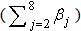.

### At a glance: Figures

123456
Prev Next

• Agarwal, Preeti, A. Ray, and A. Roy Chowdhury. "Properties of Optical Soliton in a Three Level Medium with Quintic Nonlinearity." International Journal of Physics 3.2 (2015): 45-51.
• Agarwal, P. , Ray, A. , & Chowdhury, A. R. (2015). Properties of Optical Soliton in a Three Level Medium with Quintic Nonlinearity. International Journal of Physics, 3(2), 45-51.
• Agarwal, Preeti, A. Ray, and A. Roy Chowdhury. "Properties of Optical Soliton in a Three Level Medium with Quintic Nonlinearity." International Journal of Physics 3, no. 2 (2015): 45-51.

 Import into BibTeX Import into EndNote Import into RefMan Import into RefWorks

### 1. Introduction

Solitary wave propagation has proved to be of immense use in various branches of physics where the wave phenomenon holds a very central place. The formation of a solitary wave, or the so-called soliton, is a very interesting process, which is actually an outcome of dispersion, diffraction and nonlinearity all put together . While the birth of the soliton  was in fluid mechanics, later it has got enormous applications in many other situations, one of them being nonlinear fiber optics [3, 4, 5]. But a different situation arises in atomic transitions as a result of self-induced transparency , a coupling between the electric field and the quantum states of the atom, whereby the pulse propagates undistorted and unattenuated. It is actually called Self Induced Transparency soliton [7, 8]. In recent years many works have been done on coherent propagation of light in three level medium [9, 10, 11]. These can be called Self Induced Transparency-Non Linear Schrödinger soliton [12, 13].

In this paper we report an investigation of the propagation of the soliton in a three level medium where higher order nonlinearity exists in the polarization of the electromagnetic field. Over and above, we have induced polarization due to the material transitions. We investigate the role of power of the pulse, magnitude of dispersion and nonlinearity in details and observe that the higher order nonlinear terms can be effectively compensated if all the possible dispersive terms up to the eighth order are retained. In fact this can lead to the interesting phenomenon of continuum generation.

### 2. Formulation

To analyze the propagation of two optical pulses through a doped nonlinear dispersive medium modelled by a resonant three level system, we start with the basic Hamiltonian and the state vectors of the material system. The Hamiltonian is written as[14, 15],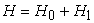(1)

where,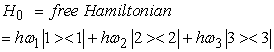(2)

where, ‘h’ is defined as the reduced Planck constant or the Dirac constant. It is equal to the Planck constant(h) divided by 2π.

The time dependent atomic wave function is,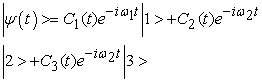(3)

The interaction picture equation governing the evolution is,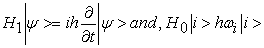where, i = 1, 2, 3. The interaction Hamiltonian H1 can be written as,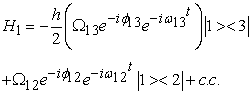(4)

where,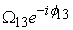and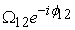are the complex Rabi frequencies associated with the field modes of frequencies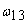and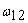, resonant with the dipole allowed transition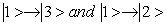respectively. Then the above equation of motion leads to,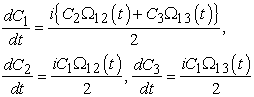(5)

where,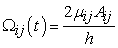.

In this case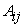’s are the slowly varying amplitudes that compose the total electric field, that is,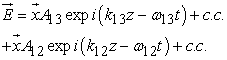(6)

where,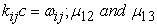are the electric dipole moments associated to transitions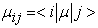. On the other hand, we treat the electromagnetic field classically, which is quite a good approximation for the present purpose. When two optical waves of different frequencies co-propagate in a medium and interact nonlinearly through the medium, the propagation equations are to be considered along with those of the material given in equations(5).

The extended Nonlinear Schrödinger type equations that describe the propagation of the envelope of our two optical fields are,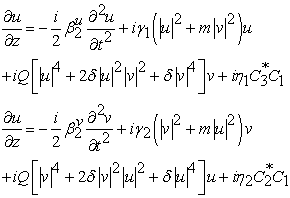(7)

If one wants to observe the effect of continuum generation, one can keep higher order dispersive terms on the right hand side of equations (7). This means that the second order dispersive term for both optical fields u and v represented as,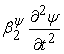, can be replaced by higher order dispersive terms,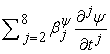, so that the coupled equations (7) may explicitly be written as,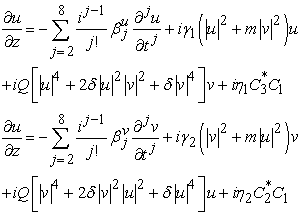where the values of the constants,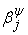, (j = 2, ....8) are to be chosen appropriately. Here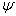is either u or v andrepresents the higher order dispersion terms, in this case, up to the eighth order.

In the above equations,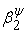represents the second order dispersion term for the pulses u and v respectively, that is,is either u or v.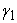and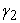represent Kerr nonlinearities,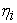’s determine the coupling to the atomic system for the ‘i’th mode (where, i = 1 or 2), Cn’s are the probability amplitudes of the atomic levels jn > for the three level atomic system within the rotating wave approximation, where n = 1, 2 and 3 respectively and Q is the coupling strength for fifth order nonlinearity. Here, m is the cross phase modulation(XPM) term, that is, this term induces a nonlinear coupling between the field components u and v. Also we have considered δ as the nonlinear coupling term between the field components u and v for the fifth order nonlinearity.

### 3. Solutions and Numerical Simulations

Now to analyze the solution of the coupled system defined by equations (5) and (7), one may adapt various techniques known but one of the most accurate one is that of split-step technique. In the usual split-step method one treats the linear and nonlinear terms separately. But here due to the terms coupled to equations (5), we are to treat equations (5) with standard fourth order Runge-Kutta method along with the symmetrized split-step method [1, 16, 17]. The actual methodology is as follows:

The split step method makes use of the fundamental property of Fourier transforms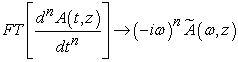where ‘FT’ denotes the Fourier Transform and ‘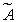’ is the Fourier transformed field. The split-step technique takes advantage of this property by treating the terms involving derivatives in Fourier space while accounting for the nonlinear terms in the space domain. The step is split between two different types of effects, one treated in the space domain and the other treated in the frequency domain.

To solve the master equations using this technique, it is instructive to write them in the form,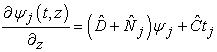where, j = 1, 2, thereby,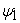= u and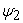= v.

The dispersive terms are given by,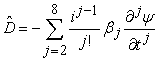where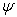is either ‘u’ or ‘v’, and the nonlinear terms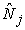are given by,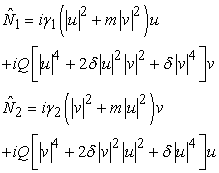The term labeled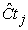(for the constant terms) are given as,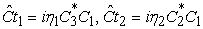In the symmetrized split-step the solution is approximated as,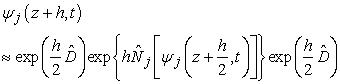To ascertain the characteristics of the pulse propagation we have to estimate the variation of chirp and spectral width along with the modulus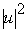and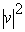. In the present analysis we have estimated these by the following fomulae :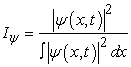(8)

for the intensity distribution wherestands for either u or v.

The root mean square spectral width (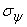) is given as,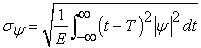(9)

where,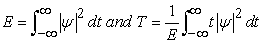The next important aspect of the pulse is its chirp. The root mean square chirp is given by,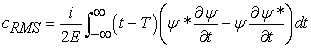(10)

In each case,stands for either u or v.

In our present scenario after the integration of the coupled system described by equations(5) and (7) we have evaluated the integrals given by equations(8) to (10) for the pulse parameters.

Figure 1. Propagation of bright-bright pulses shown in figures labelled (c) and (d) respectively with their corresponding intensity profiles shown by figures (a) and (b) respectively.

It may further be added that the coupled system has two classes of solutions given by,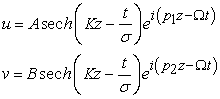which, physically speaking, corresponds to the case of bright-bright pulse and the solution below corresponds to bright-dark pulse propagations given by,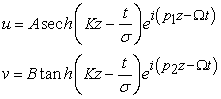Figure 2. Same as in Figure 1 for bright-dark pulses shown by labels (a) and (b) with the corresponding profiles shown by figures (c) and (d) respectively

In our above numerical simulations we have considered both the situations. Let us elaborate on each case in detail.

In Figure 1a and Figure 1b we have shown the nature of the pulse propagation for the bright-bright case whereas in Figure 2a and Figure 2b we show the bright dark situation. In each case, we have considered the usual dispersive situation and the special case of continuum generation, when all the eight terms of higher order dispersion are considered.

From our results it may be seen that the forms of the pulses remain much better in the latter case. The spectral widths as calculated from the formulae given by equation (9) are displayed in Figure 3. It clearly shows the variation due to the presence of higher order dispersive terms.

In our analysis in Figure 3, we have shown the spectral width for the bright bright case involving the quintic term. In the figure, we show the comparison of the spectral width considering second order dispersion (β2) depicted in Figure 3c and Figure 3d with the spectral width obtained by keeping higher order dispersion terms (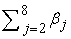) shown in Figure 3a and Figure 3b. We see clearly that the width is more stable and better-oriented in Figure 3a and Figure 3b where we have considered the case of supercontinuum generation.

In Figure 4 we have shown the same case for chirp based on similar criterion. The figures again show better stability when the effects of higher order terms of dispersion are also included.

Figure 3. Spectral width of bright-bright pulses shown in figures marked by (a) and (b) respectively considering all the β values while figures marked by (c) and (d) respectively show the same for only β2 values. The values of βj’s in (a) and (b) are considered as, -9.5205 X 10-8fs2/mm, 2.0737 X 10-10fs3/mm, -5.3943 X 10-13fs4/mm, 1.3486 X 10-15fs5/mm, -2.5495 X 10-18fs6/mm, 3.0524 X 10-21fs7/mm and -1.7140 X 10-24fs8/mm, where, j = 2, ..., 8, whereas, β2 in (c) and (d) is considered to be -9.5205 X 10-8fs2/mm.
Figure 4. Chirp of bright-bright pulses shown in figures marked by (a) and (b) respectively considering all the β values while figures marked by (c) and (d) respectively show the same for only β2 values. The values of β and β2 are taken the same throughout as written earlier.
Figure 7. Spectral width variation for bright-bright pulse (i) in presence of quintic terms shown by figures labelled (a) and (b) and (ii) in absence of quintic terms shown by figures (c) and (d). Here we consider only the second-order dispersion (β2)

Also we have analyzed the bright-dark case for the above coupled equations which are shown in Figure 5 and Figure 6 respectively for the width and the chirp.

Figure 8. Variation of chirp for sech-sech type pulse in the presence of second-order dispersion (β2) only. Figures (a) and (b) show the variation in presence of the quintic terms and figures (c) and (d) show the variation in the absence of the quintic terms

Here also, both the figures show a better behaviour and remarkable stability when the higher order dispersion terms () are considered.

Now let us compare the quintic and the non-quintic cases. We have shown the effect on spectral width with the presence and absence of quintic terms for the sechsech pulse profile considering higher orders of dispersion (the case of super continuum generation). This has been shown in Figure 9 where the variations are again much pronounced if we do not consider the quintic terms (as shown in Figure 9c and Figure 9d) and the situation is much better in Figure 9a and Figure 9b where the higher order terms of dispersion are also considered. The same situation is revealed in Figure 13 where we have considered the sech-tanh type pulse.

A similar analysis has also been done for the case of chirp associated with the pulse.

Let us now compare the situation when the quintic terms are absent. We first consider the situation when only second-order dispersion (β2) is present as shown in Figure 7c and Figure 7d. We have also shown the plots of spectral width in Figure 7a and Figure 7b for the situation where the quintic terms are present. The comparison reveals clearly that the situation is better and much more stable when the quintic terms are considered whereas the variations are much pronounced when quintic terms are absent. This is the situation when both the pulses are considered to be of sech type.

We have also analysed a similar situation shown in Figure 11 for the bright-dark pulses. This plot also reveals the better stability and orientation of the pulses when we consider the quintic terms as shown in Figure 11a and Figure 11b rather than when these terms are absent as shown in Figure 11c and Figure 11d.

Figure 8 shows the comparison of the quintic effect in case of bright-bright pulse while considering only second order dispersion. The same analysis is shown in Figure 10 where higher order dispersion terms () are considered instead of β2 only. In Figure 12 and Figure 14 we consider the case for sech-tanh pulse. Figure 12 shows the situation for β2 only, when the quintic terms are present (Figure 12a and Figure 12b) and when there are no quintic terms(Figure 12c and Figure 12d). Retaining the case of sech-tanh pulse we show the variation of chirp for higher order dispersion terms () in Figure 14. Figure 14a and Figure 14b show the situations when the quintic terms are present and Figure 14c and Figure 14d deal with the cases where no quintic term is present.

Lastly we have plotted the difference of values for spectral width and chirp obtained with or without the “quintic” term to emphasize the effect of higher order nonlinearity. These are exhibited in Figure 15 and Figure 16, whereby in Figure 15 we have plotted the difference values considering the higher order values of dispersion () and in Figure 16 we have plotted the difference values considering the second order dispersion (β2) only. These figures are plotted for sech-sech type pulses. The figures clearly exhibit better approach in presence of higher order dispersion terms.

Similar plots can also be drawn for sech-tanh type pulse.

Figure 9. Same as in Figure 7 but here, in addition to the second-order dispersion term, we consider higher order terms of dispersion also, i.e., ()
Figure 10. Same as in Figure 8 considered for higher order terms of dispersion, i.e., ()
Figure 15. Difference graph plotted for spectral width and chirp in the presence and absence of quintic terms for sech-sech type pulse. Here we have considered higher order dispersion terms ()
Figure 16. Difference graph plotted for spectral width and chirp in the presence and absence of quintic terms for sech-sech type pulse. Here we have considered second order dispersion (β2) only

### 4. Conclusion

In our above analysis we have studied the mutual influence of the material induced polarization, higher order nonlinearity and dark-bright soliton interaction on the pulse propagation in a three level medium. The situations of standard dispersion and higher order dispersions are considered. It is observed that in the latter case we have better propagation characteristics. On the other hand, the coupling of the material medium also has a strong effect on the pulse shaping. We have investigated both the effect of higher order dispersions and higher order nonlinearity on the optical pulse propagation in a three level medium, when the medium is treated quantum mechanically and the light propagation classically. The interplay between these two effects is very interesting from the viewpoint of actual fabrication.

### Acknowledgement

One of the authors (A.R.C.) is grateful to U.G.C. (Govt. of India) for a BSR fellowship and A. R. is grateful to C.S.I.R. for a senior research fellowship which made this work possible.

### References

  G. P. Agarwal, Nonlinear Fiber Optics, 2nd Ed., New York Academic Press, 1995.In article  J. S. Russell, “Report on waves”. Fourteenth meeting of the British Association for the Advancement of Science, 1844.In article  A. Hasegawa and F. Tappert, Appl. Phys. Lett., 23 (3) 1973 142.In article  A. Hasegawa and F. Tappert, Appl. Phys. Lett., 23 (4) 1973 171.In article  L.F. Mollenauer, R.H. Stolen, and J.P. Gordon, Phys. Rev. Letters, 45 (13) (1980) 1095.In article  S. L. McCall and E. L. Hahn, Physical Review Lett., 183 (2) (1969) 457.In article  T. N. Dey, S. D. Gupta and G. S. Agarwal, Optics Express, 16 (22) (2008) 17441.In article  N. P. Bigelow, J. H. Eberly and C. R. Stroud Jr., Coherence and Quantum Optics VIII, 2003.In article  J. H. Eberly, Quantum Semiclass. Opt., 7 (1995) 373.In article  A. I. Maimistov and E. A. Manykin, Zh. Eksp. Teor. Fiz., 85, (1983) 1177.In article  I. V. Mel'nikov, R. F. Nabiev and A. V. Nazarkin, Opt. Lett., 15 (1990) 1348.In article  S. B. Cavalcanti, E. J. S. Fonseca, D. P. Caetano and J. M. Hickmann, An. Acad. Bras. Cienc., 73 (2001) 197.In article  E. J. S. Fonseca, S. B. Cavalcanti and J. M. Hickmann, Annals of Optics-XXV ENFMC, (2002) 81.In article  A. I. Maimistov and E. A. Manykin, Zh. Eksp. Teor. Fiz., 85, (1983) 685.In article  M. Nakazawa, E. Yamada and H. Kubota, Phys. Rev. Lett., 66 (1991) 2625.In article  O. V. Sinkin, R. Holzlöhner, J. Zweck and C. R. Menyuk, Journal of Lightwave Technology, 21 (2003) 61.In article  S. Balac and F. Mah´e, Journal of Computational Physics, 280 (2015) 295.In article  B. Mandal and A. R. Chowdhury, Chaos, Solitons and Fractals, 24 (2005) 557.In article